This guide covers all the geometry you need for the GRE math section. We will try to make the content fairly intuitive, combining formulas with some explanation of why the formula makes sense. Doing well on geometry problems can make a big difference for your GRE math score since geometry questions make up about 15% of all GRE math questions.

Most geometry questions will ask you to do one of four things:

1. Find the line length
Example 1
In the following figure, find the value of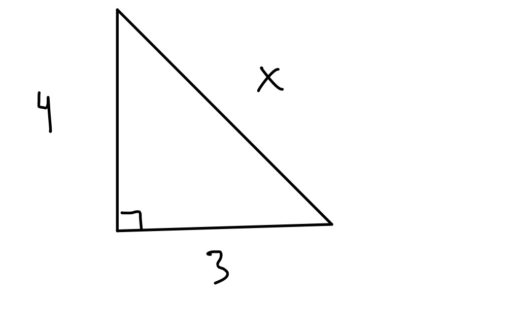2. Find the angle
Example 2
Find the value of: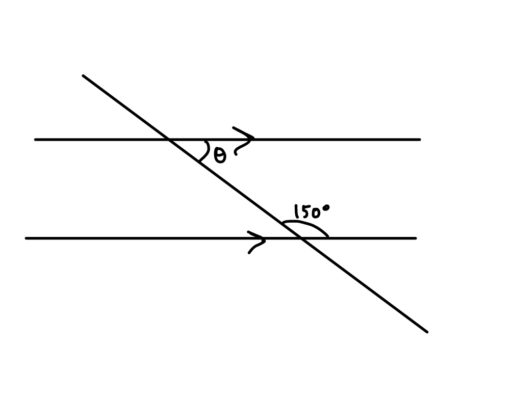3. Find the area (of a 2D shape) or surface area (of a 3D shape), or
Example 3
Find the area of the shaded region:4. Find the volume (of a 3D shape)
Example 4
Find the volume of the rectangular prism below: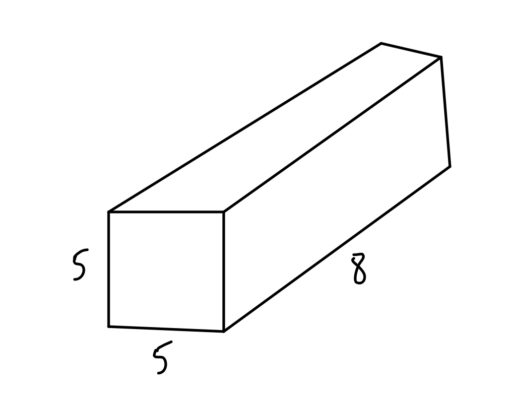But these problems can be difficult because they give you seemingly odd bits of information. For example, they might ask:

Example 5
The area of the following triangle is 20. Find: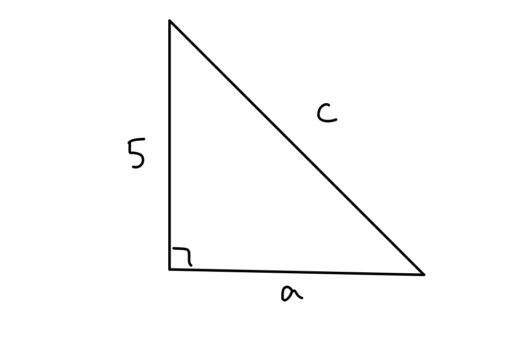and you will have to figure out a way to use what you know (the area formula for triangles, the Pythagorean Theorem) to figure out the answer:

There are many little factoids which matter in geometry, and these will be found throughout our guide. Now, the sheer quantity of formulae can get overwhelming, so we will distinguish between must-know formulas like:

Pythagorean Theorem: For any right triangle whereare the legs of the triangle andis the hypotenuse,and the less critical formulas like:

30-60-90 Right Triangle: For any right triangle with angles of 30, 60, and 90 degrees, the side lengths have the following ratio: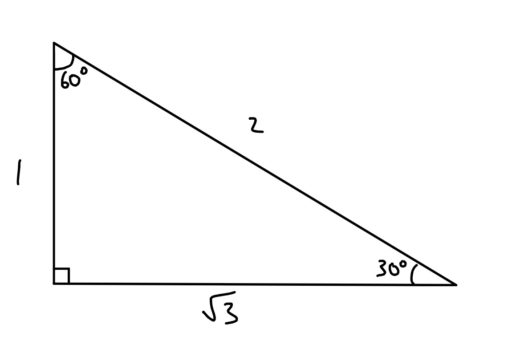The must-know formulas are, well, a must-know if you want to do well on geometry. But if you are okay with missing a few geometry questions on the exam, you can afford to ignore some of the less critical formulas. Each such formula comes up maybe once per test.